### Home > CCG > Chapter 3 > Lesson 3.1.4 > Problem3-41

3-41.

Solve for the missing lengths in the sets of similar figures below.

1. $ΔABC\simΔOPQ$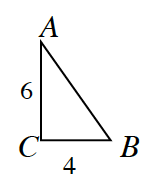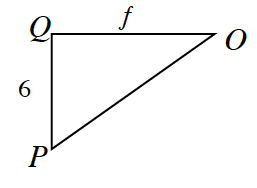$\frac{6}{f} = \frac{4}{6}$

$f=9$

2. $EFGHI\sim STUVW$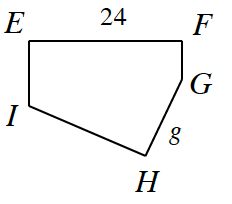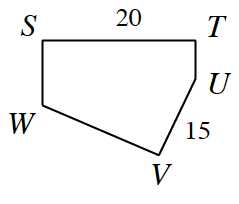$\frac{24}{20} = \frac{g}{15}$

3. $JKLM ∼ WXYZ$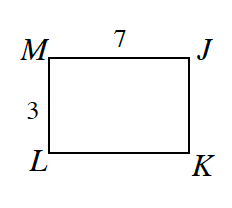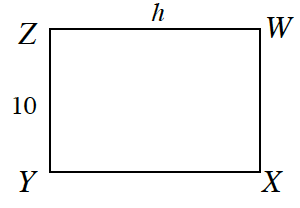Use the same method you used to solve parts (a) and (b).